打造自己的LINQ Provider（上）：Expression Tree揭秘

认识表达式目录树

Expression<Func<int, int, int>> expression = (a, b) => a * b + 2;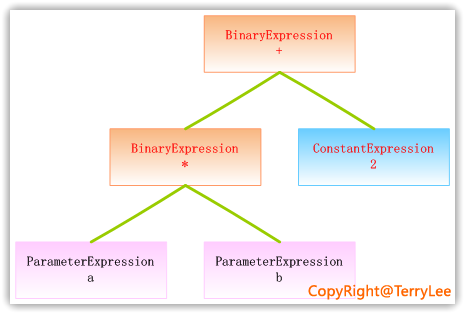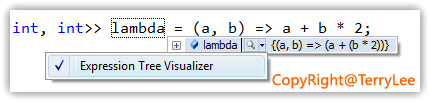static void Main(string[] args)
{
ParameterExpression paraLeft = Expression.Parameter(typeof(int), "a");
ParameterExpression paraRight = Expression.Parameter(typeof(int), "b");

BinaryExpression binaryLeft = Expression.Multiply(paraLeft, paraRight);
ConstantExpression conRight = Expression.Constant(2, typeof(int));

LambdaExpression lambda =
Expression.Lambda<Func<int, int, int>>(binaryBody, paraLeft, paraRight);

Console.WriteLine(lambda.ToString());

}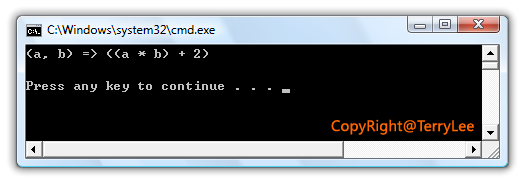Body：指表达式的主体部分；

Parameters：指表达式的参数；

NodeType：指表达式的节点类型，如在上面的例子中，它的节点类型是Lambda；

Type：指表达式的静态类型，在上面的例子中，Type为Fun<int,int,int>。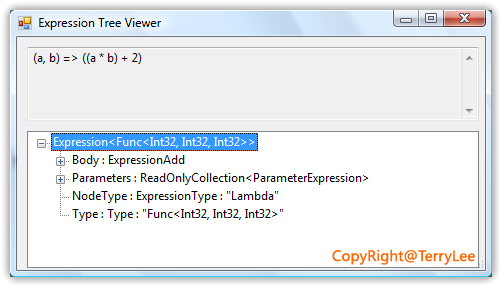表达式目录树与委托

static void Main(string[] args)
{
Func<int, int, int> lambda = (a, b) => a + b * 2;

Expression<Func<int, int, int>> expression = (a, b) => a + b * 2;
} 

.method private hidebysig static void  Main(string[] args) cil managed
{
.entrypoint
.maxstack  3
.locals init ( class [System.Core]System.Func3<int32,int32,int32> lambda)
IL_0000:  nop
IL_0001:  ldsfld     class [System.Core]System.Func3<int32,int32,int32>
TerryLee.LinqToLiveSearch.Program::'CS$<>9__CachedAnonymousMethodDelegate1' IL_0006: brtrue.s IL_001b IL_0008: ldnull IL_0009: ldftn int32 TerryLee.LinqToLiveSearch.Program::'<Main>b__0'(int32, int32) IL_000f: newobj instance void class [System.Core]System.Func3<int32,int32,int32>::.ctor(object, native int) IL_0014: stsfld class [System.Core]System.Func3<int32,int32,int32> TerryLee.LinqToLiveSearch.Program::'CS$<>9__CachedAnonymousMethodDelegate1'
IL_0019:  br.s       IL_001b
IL_001b:  ldsfld     class [System.Core]System.Func3<int32,int32,int32>
TerryLee.LinqToLiveSearch.Program::'CS$<>9__CachedAnonymousMethodDelegate1' IL_0020: stloc.0 IL_0021: ret } 而第二句，由于告诉编译器是一个表达式目录树，所以编译器会分析该Lambda表达式，并生成表示该Lambda表达式的表达式目录树，即它与我们手工创建表达式目录树所生成的IL是一致的，如下代码所示，此处为了节省空间省略掉了部分代码： .method private hidebysig static void Main(string[] args) cil managed { .entrypoint .maxstack 4 .locals init ( class [System.Core]System.Linq.Expressions.Expression1< class [System.Core]System.Func3<int32,int32,int32>> expression,  class [System.Core]System.Linq.Expressions.ParameterExpression CS$0$0000,  class [System.Core]System.Linq.Expressions.ParameterExpression CS$0$0001,  class [System.Core]System.Linq.Expressions.ParameterExpression[] CS$0\$0002)
IL_0000:  nop
IL_0001:  ldtoken    [mscorlib]System.Int32
IL_0006:  call       class [mscorlib]System.Type [mscorlib]System.Type::GetTypeFromHandle(...)
IL_000b:  ldstr      "a"
IL_0010:  call       class [System.Core]System.Linq.Expressions.ParameterExpression
[System.Core]System.Linq.Expressions.Expression::Parameter(
class [mscorlib]System.Type,

IL_0038:  call    class [mscorlib]System.Type [mscorlib]System.Type::GetTypeFromHandle()
IL_003d:  call    class [System.Core]System.Linq.Expressions.ConstantExpression
[System.Core]System.Linq.Expressions.Expression::Constant(object,
class [mscorlib]System.Type)
IL_0042:  call    class [System.Core]System.Linq.Expressions.BinaryExpression
[System.Core]System.Linq.Expressions.Expression::Multiply(class [System.Core]System.Linq.Expressions.Expression,
class [System.Core]System.Linq.Expressions.Expression)
IL_0047:  call    class [System.Core]System.Linq.Expressions.BinaryExpression
class [System.Core]System.Linq.Expressions.Expression)
IL_004c:  ldc.i4.2
IL_004d:  newarr     [System.Core]System.Linq.Expressions.ParameterExpression
}

执行表达式目录树

static void Main(string[] args)
{
ParameterExpression paraLeft = Expression.Parameter(typeof(int), "a");
ParameterExpression paraRight = Expression.Parameter(typeof(int), "b");

BinaryExpression binaryLeft = Expression.Multiply(paraLeft, paraRight);
ConstantExpression conRight = Expression.Constant(2, typeof(int));

Expression<Func<int, int, int>> lambda =
Expression.Lambda<Func<int, int, int>>(binaryBody, paraLeft, paraRight);

Func<int, int, int> myLambda = lambda.Compile();

int result = myLambda(2, 3);
Console.WriteLine("result:" + result.ToString());

}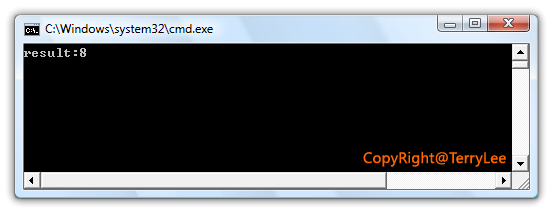static void Main(string[] args)
{
Expression.Constant(2),
Expression.Constant(3));

Expression<Func<int>> expression =
Expression.Lambda<Func<int>>(body, null);

Func<int> lambda = expression.Compile();

Console.WriteLine(lambda());
}

访问与修改表达式目录树

static void Main(string[] args)
{
Expression<Func<int, int, int>> lambda = (a, b) => a + b * 2;

Console.WriteLine(lambda.ToString());
} 

public class OperationsVisitor : ExpressionVisitor
{
public Expression Modify(Expression expression)
{
return Visit(expression);
}

protected override Expression VisitBinary(BinaryExpression b)
{
{
Expression left = this.Visit(b.Left);
Expression right = this.Visit(b.Right);
return Expression.Subtract(left,right);
}

return base.VisitBinary(b);
}
}

static void Main(string[] args)
{
Expression<Func<int, int, int>> lambda = (a, b) => a + b * 2;

var operationsVisitor = new OperationsVisitor();
Expression modifyExpression = operationsVisitor.Modify(lambda);

Console.WriteLine(modifyExpression.ToString());
}`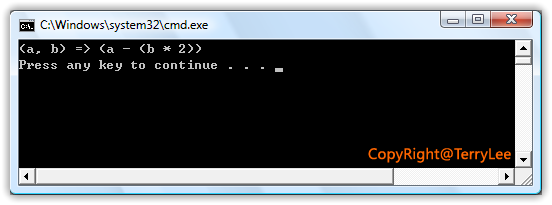为什么需要表达式目录树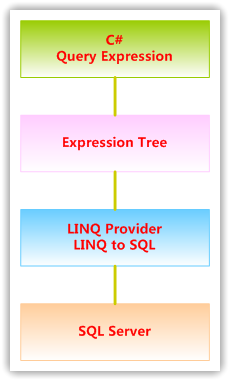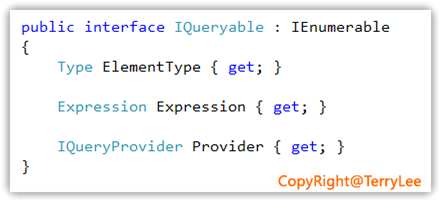总结

posted @ 2008-08-01 01:19 TerryLee 阅读(...) 评论(...) 编辑 收藏